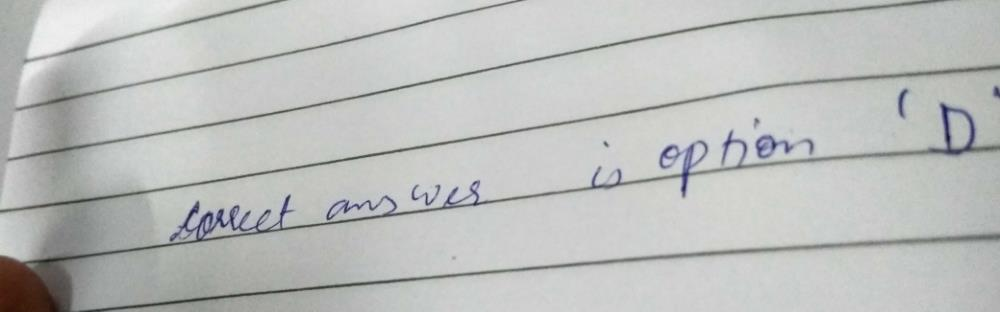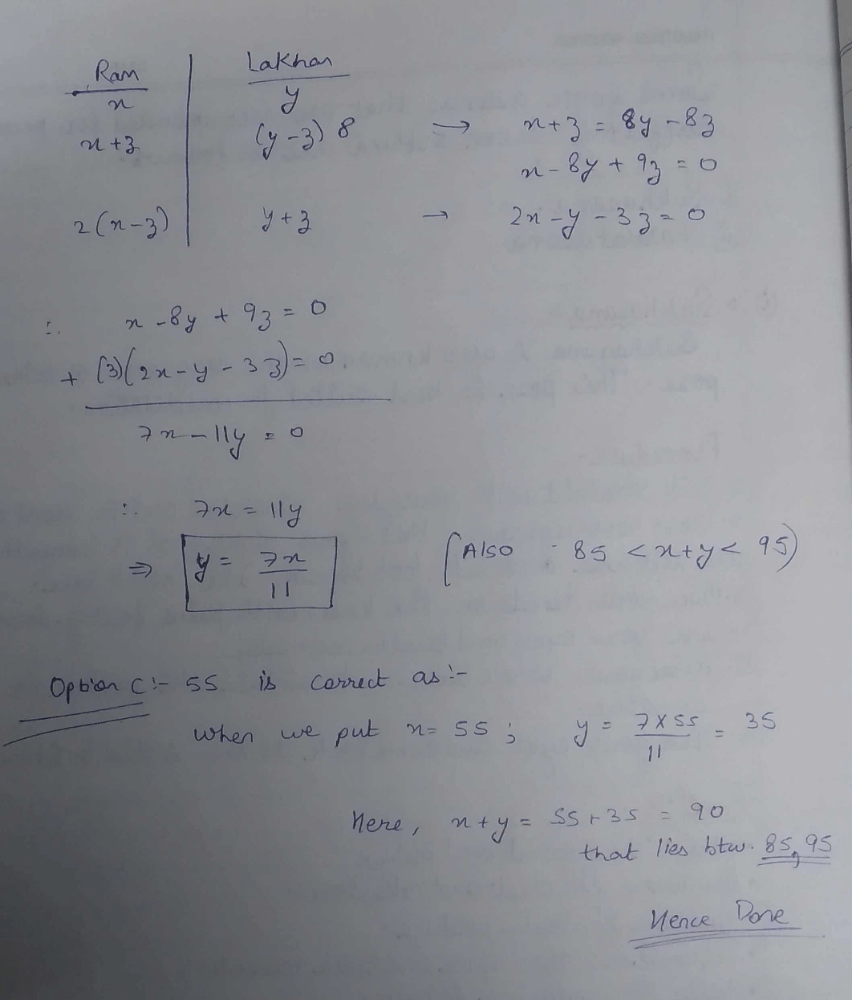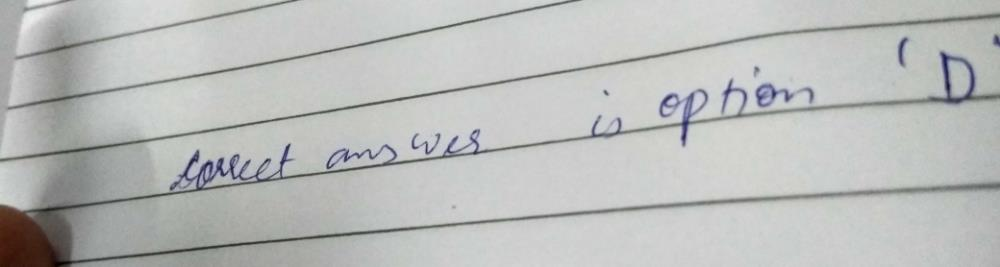Courses

# The total number of chocolates with Ram and Lakhan is more than 85 but less than 95. If Lakhan gives a particular number of chocolates to Ram, then Ram will have eight times the number of chocolates left with Lakhan and if Ram gives the same number of chocolates to Lakhan, then Lakhan will have double the number of chocolates left with Ram. How many chocolates does Ram have? a)44 b)50c)55d)56e)Cannot be determinedCorrect answer is option 'C'. Can you explain this answer? Related Test: NMAT Quantitative Skills MCQ Quiz - 1

## CAT Question

By Dhiren Mandowara · Dec 29, 2019 ·CAT
Vishwanathan K answered Feb 22, 2020
The total number of chocolates has to lie between 85 and 95 and has to be divisible by 8+1=9 as well as 2+1=3. The only number satisfying these is 90.
Result I: Ram 80, Lakhan 10
Result II: Ram 30, Lakhan 60
Number of chocolates with Ram = (80+30)/2 = 55
Number of chocolates given/taken by Ram = (80-55) = (55-30) = 25

Patcha Vinay answered Dec 01, 2019
Le X be intially no of chocolates with ram and y be no of chocolates with lakhan.
X+n=8(y-n):x-8y=-9n,2x-y=3n,y=1.4n,X=2.2n:8595: 85<3.6n>95;either n=24,25,26.x and y are integers so 1.4n is integer n=25;ram have x=2.2n=55

Kamlesh Mukesh answered Jun 09, 2020
Hii

Abhay Chabbra answered Feb 20, 2020
A

Mamata Das answered Jul 10, 2020
D. 56

Ankit Sharma answered Feb 28, 2020
B

Jane Mukiri answered May 23, 2020
C

Priya Tiwari answered Jun 23, 2020
A/q 85 < />< />
let X chocolate L gives to R then
(L- X)8 = R+X
8L-R=9X
same chocolate R gives to L
2( R- X )=L+X
2R-L=3X
now , multiple by 2 in equ 1and then add with equ 2
then we find that,
L=7X/5 and R=11X/5
we take The value of X is 5 10 15 20 25 more than this value total exceed from 95 so we can't take
while we take the value of X is 25 condition will satisfied
so R will have=11*25/5=55
L have=7*25/5=35
and sum of both is 55+35=90 , which is full fill my all conditions

Vishal Agarwal answered May 06, 2020
Let the number of chocolates with Lakhan be x and the number of chocolates with Ram be y
Now, Let Lakhan give Ram z number of chocolates
Therefore, Lakhan has now = x-z & Ram has now = y+z
Now Ram would have 8 times the number of chocolates left with Lakhan which is = 8(x-z)
According to the problem,
8(x-z) = y+z
or, 8x-8z=y+z
or, 8x-y= 8z+z
or, 8x-y= 9z - Equation(1)
Again, Ram gives the same number of chocolates to Lakhan which is z number of chocolates
Therefore, Lakhan has now = x+z & Ram has now = y-z
Now Lakhan would have double the number of chocolates left with Ram which is = 2(y-z)
According to the problem,
x+z= 2(y-z)
or, x+z= 2y-2z
or, 2y-x=z+2z
or, 2y-x= 3z - Equation(2)
Now, by dividing Equation (1) by Equation (2), we get,
9z/3z=8x-y/2y-x
or, 8x-y/2y-x=3
or, 8x-y=3(2y-x)
or, 8x-y=6y-3x
or, 8x+3x=6y+y
or, 11x=7y
or, x=7y/11
Total number of chocolates with Ram and Lakhan = x+y = 7y/11+ y = 18y/11
when Total is more than 85 which is
85<18y>
or, y>(85*11) /18
or, y>52
when Total is less than 95
18y/11< />
or, y<(95*11)>
or, y< />
Therefore, 52< />< />
Accordingly, y should be between 52 & 58
Thus, y could either be 55 or 56
Thus, the correct answer could either be C or D
THANK YOU

Keshav Kaushik answered Apr 13, 2020
Let he gave d chocolates to other person and vice versa.
r + d = 8 (l-d)
2(r-d) = l+d
solve for 7r=11l

now 85< 18r/11="" />< />
for r = 55 only this condition is satisfied (integer).

Ankul Lohiya answered Jun 14, 2020
The total number of chocolates lies between 85 to 95..
so no. of chocolates should be divisible by 8+1=9, and 2+1=3...
then the number is only 90, which divisible by both numbers 9&3,
so Ram have 55 and lakhan have 25 chocolates..Raksha Saxena answered 2 weeks agoRishab Bhatnagar answered May 22, 2020